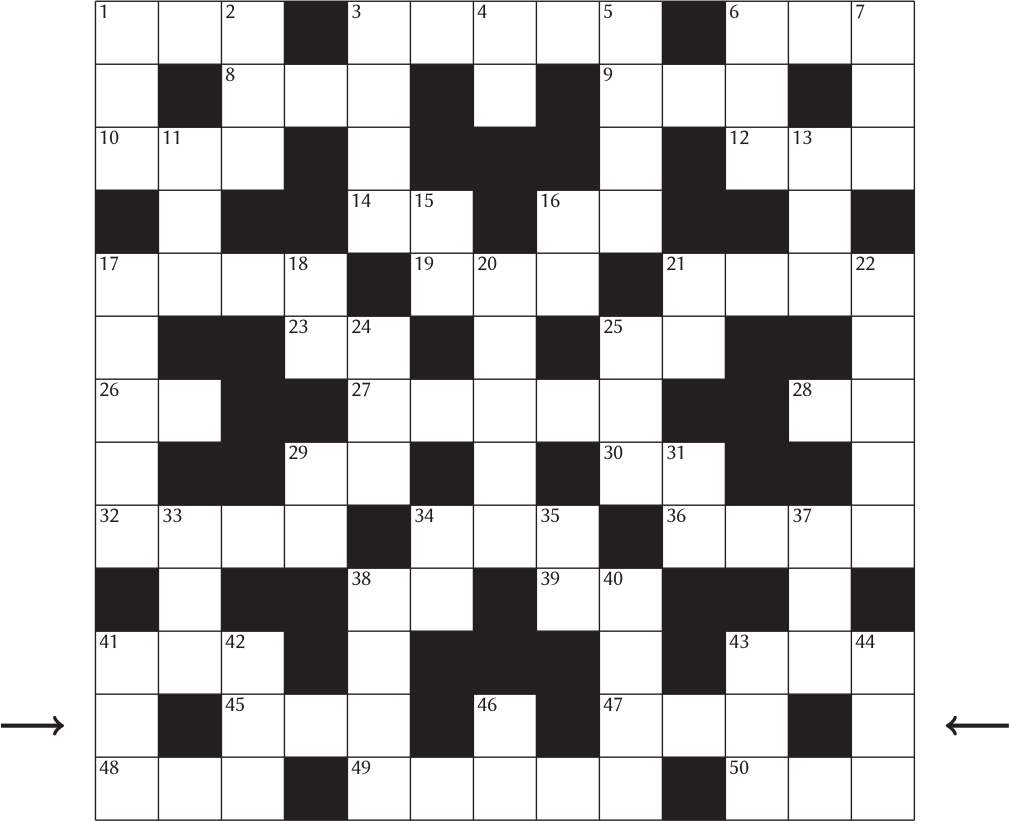# Prize crossnumber, Issue 16

Can you solve it?Our original prize crossnumber is featured on pages 36 and 37 of Issue 16.

### Corrections

• There was a typo in the clue for 1D: This originally read “One less than 1D”, when it should have been “One less than 1A.. This has been corrected below and in the pdf linked above.

### Rules

• Four of the across clues and four of the down clues are false. All the other clues are true.
• Solvers may wish to use the OEIS, Wikipedia, Python, a slide rule, etc to (for example) obtain a list of cube numbers, but no programming should be necessary to solve the puzzle. As usual, no numbers begin with 0.
• One randomly selected correct answer will win a £100 Maths Gear goody bag, including non-transitive dice, a Festival of the Spoken Nerd DVD, and much, much more. Three randomly selected runners up will win a Chalkdust T-shirt. Maths Gear is a website that sells nerdy things worldwide, with free UK shipping.
• To enter, submit the sum of all the digits in the row marked by arrows using this form by 14 April 2023. Only one entry per person will be accepted. Winners will be notified by email and announced on our blog by 1 May 2023.

### Crossnumber

Crossnumber #16, set by Humbug:### Across clues

(four of these clues are false)

• 1. One less than 1D. (3)
• 3. A multiple of 11111. (5)
• 6. Each digit of this number (excluding the first) is one less than the previous digit. (3)
• 8. A multiple of 111. (3)
• 9. 111 more than 8A. (3)
• 10. The highest common factor of 48A and this number is 6. (3)
• 12. An even number. (3)
• 14. Two times 4D. (2)
• 16. A factor of 19A. (2)
• 17. The sum of this number’s digits is 11. (4)
• 19. Greater than 1A. (3)
• 21. The sum of this number’s digits is 13. (4)
• 23. A square number. (2)
• 25. One quarter of 23A. (2)
• 26. Two times 28A. (2)
• 27. The product of 24D and 25D. (5)
• 28. Not a prime number. (2)
• 29. Each digit of this number is a factor of 38D. (2)
• 30. One quarter of 29A. (2)
• 32. An anagram of 17A. (4)
• 34. 15 less than 10A. (3)
• 36. An anagram of 21A. (4)
• 38. A multiple of 3 whose final digit is the same as the final digit of 12A. (2)
• 39. A square number. (2)
• 41. Two times 41D. (3)
• 43. The sum of this number’s digits is 10. (3)
• 45. Two times 47A. (3)
• 47. A multiple of 9. (3)
• 48. Two times 42D. (3)
• 49. A multiple of 11111. (5)
• 50. A multiple of 55. (3)

### Down clues

(four of these clues are false)

• 1. One less than 1A. (3)
• 2. Two times 10A. (3)
• 3. Two times 38D. (4)
• 4. A factor of 1A. (2)
• 5. Two times 1D. (4)
• 6. Two times 7D. (3)
• 7. A prime number. (3)
• 11. Two times 13D. (3)
• 13. A multiple of 111. (3)
• 15. Two times 14A. (2)
• 16. A number with four distinct prime factors. (2)
• 17. A multiple of 11111. (5)
• 18. A square number. (2)
• 20. The product of 19A and 34A. (5)
• 21. Five more than 29D. (2)
• 22. A multiple of 11111. (5)
• 24. 30 more than than 6D. (3)
• 25. A prime number. (3)
• 29. Five more than 21D. (2)
• 31. A factor of 25D. (2)
• 33. Two times 11D. (3)
• 34. A factor of an anagram of 6D. (2)
• 35. Greater than 15D. (2)
• 37. Two times 33D. (3)
• 38. Two times 3D. (4)
• 40. A multiple of 1111. (4)
• 41. Two times 48A. (3)
• 42. Two times 41A. (3)
• 43. A multiple of 55. (3)
• 44. A number less than 200. (3)
• 46. The sum of the digits in the row marked by arrows. (2)Matthew is a postdoctoral researcher at University College London. He hasn’t had time to play Klax since the noughties, but he’s pretty sure that Coke is it!